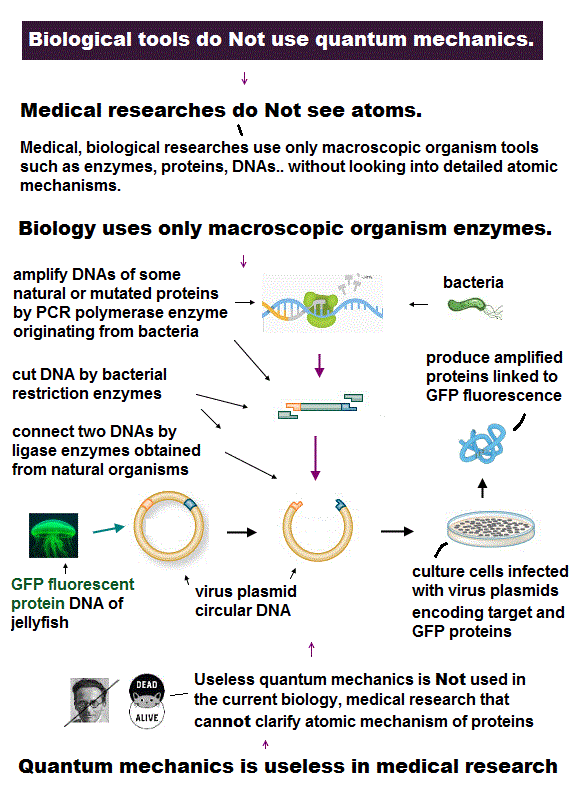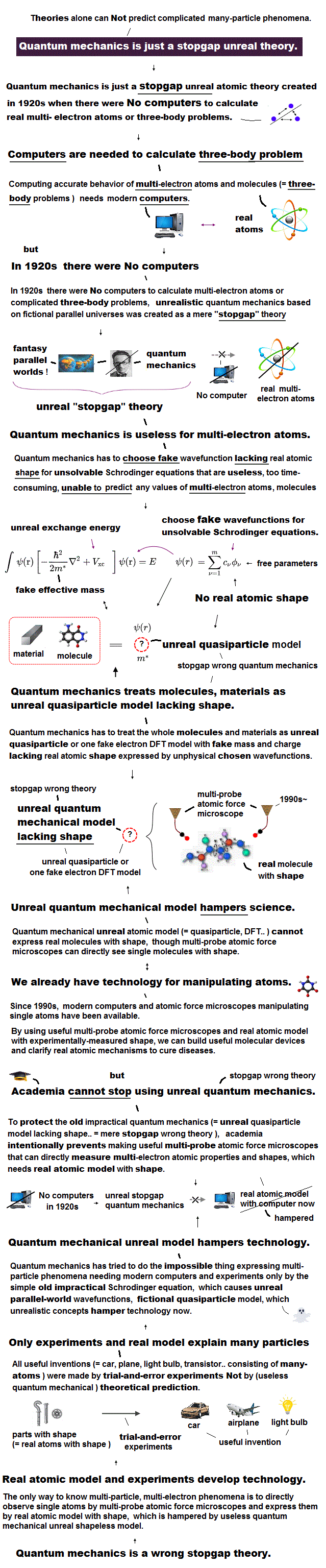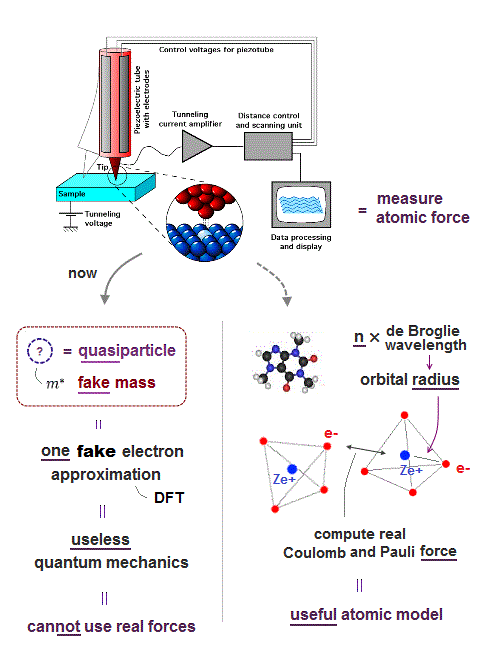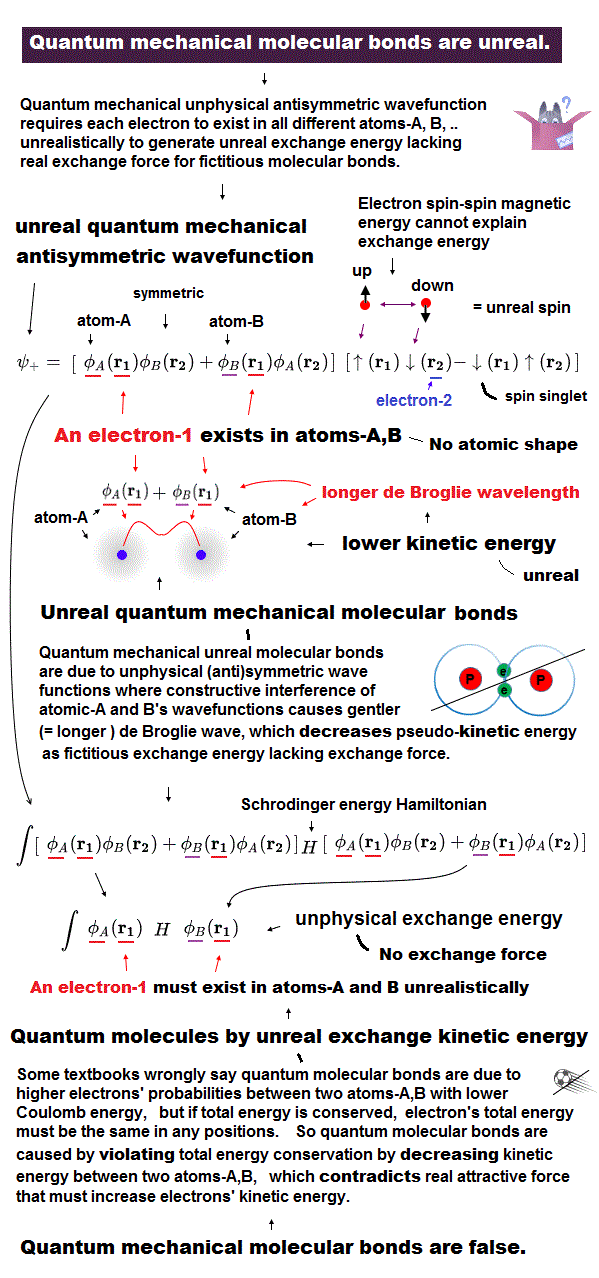﻿ Atomic tweezers by interatomic force

# Atomic tweezers control a single atom

## We can manipulate each single atom !

### [ Atomic force microscope can detect each atom and molecule ! ]

(Fig.1)  ↓ This microscope can observe and manipulate each single atom.Using atomic force microscopy, we can observe and manipulate each single atom.  So we have a cutting-edge technology to control the smallest particle.

In spite of these useful technologies, why cannot we manipulate each atomic behavior to cure fatal diseases such as cancer and HIV ?

In fact, old basic theory prevents us from advancing !

## Atomic tweezers can pick up a single molecule.

### [ Quantum mechanics prevents us from using atomic tweezers ! ]

(Fig.2)  "Mechanical force" atmong atoms can pick up a single atom.Like machanical force among things, even atoms repel each other as their distance is closer.  These forces can be measured by atominc force microspopy.

Using these repulsive forces among atoms, we can make "atomic tweezers" which can pick up a single molecule.  ( this is different ).

Realizing atomic tweezers controlling each single atom means we can make any artificial nano-molecules and enzymes to cure fatal diseases.

But in the current science, old quantum mechanics (= Schrödinger equation ) is the only option to deal with atomic interaction.

The problem is this quantum mechanics has NO realistic concepts such as "force" and "moving electrons".  It makes atomic tweezers impossible.

Its "vague" electron cloud models prevent us from formulating and using real atomic forces !

## Quantum mechanics has NO real "force" !

### [ Schrödinger equation cannot formulate atomic force. ]

(Fig.3)  Quantum mechanics cannot deal with multi-electon atoms.To realize atomic tweezers controlling a single atom, the first thing to do is formulate each atomic force.

But quantum mechanical wave function cannot define actual force, because it does not admit electron's mechanical motion.

Instead, quantum mechanics argues each single electron exists in many places at the same time in parallel worlds !  ← nonsense.

Now, Schrödinger equation is the only option for deal with interatomic behavior.  But it has NO exact solution in multi-electron atoms.

All they can do is choose fake trial function ( this p.2 ) as multi-electron solution.  We can choose any form of fake solution from infinite choices.

So quantum mechanics cannot predict multi-electron atomic behavior.

They say Pauli exclusion repulsion is caused by antisymmetric wave functions.  But they cannot find those exact wave function in multi-electron atoms.

As a result, quantum mechanics has NO ability to formulate real force using its (fake) wave functions and Pauli exclusion.

## "Real" atomic model is necessary.

### [  ① Introduce real atomic model → ② formulate atomic force  ]

(Fig.4)  Introducing real electrons' motion, real de Broglie wave is essential.We can measure each atomic force using atomic force microscope, but quantum mechanics cannot formulate these forces using 'concrete' models !

The first thing to do is introduce real and simple atomic models.  Quantum useless wave function cannot separate each single electron.

So in Schrödinger equation, two electrons cannot avoid each other by Coulomb repulsions in the simple way, which makes it very hard to formulate atomic force.

Not only Coulomb forces but also de Broglie wave is necessary to estimate each atomic radius ( atomic radius is longer as 1, 2, 3 × .. de Broglie wavelength ).

Interference among electrons' de Broglie waves can explain well about Pauli exclusion force and valence electron number.

Quantum Schrödinger equation does NOT admit real de Broglie wave, so it cannot estimate actual atomic radius.

So under vague quantum wave function (= fake solution in multi-electrons ), it's impossible to formulate actual interatomic force and atomic tweezers.2017/3/10 updated. Feel free to link to this site.